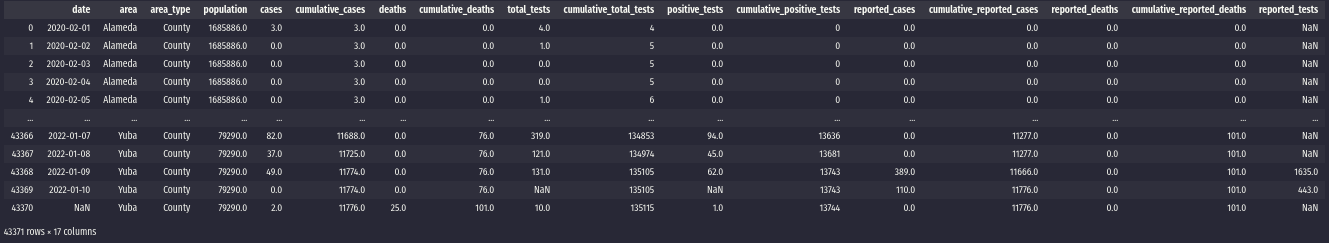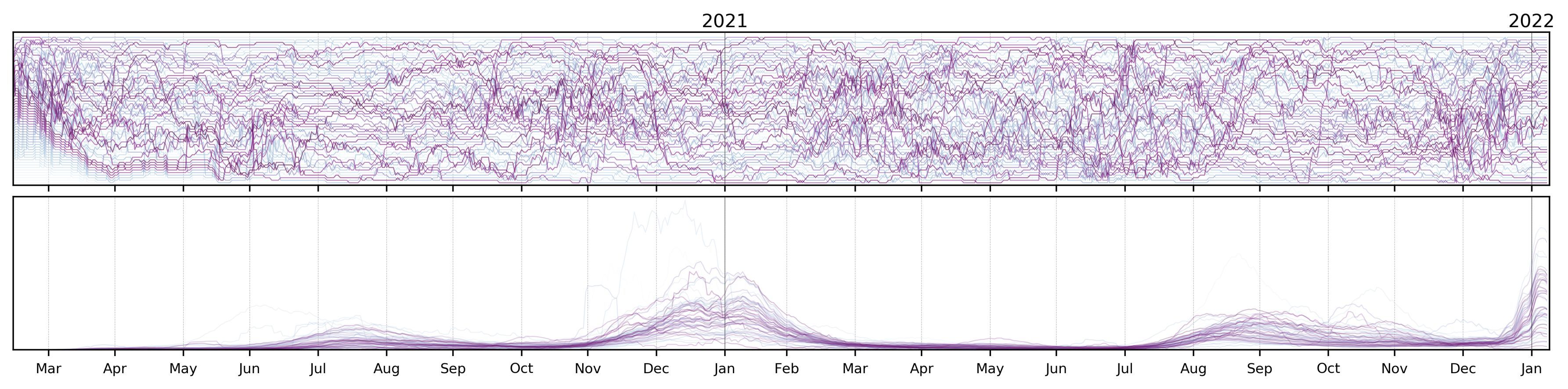# California COVID by County

Creating COVID rank-plots for counties in California.

Creating COVID rank-plots for counties in California.

# Intro

One of my friends works in the California Public Health Department and sent me the link to the COVID cases datasets for the state. I’d been wanting to do some “rank plots” for a while, so I figured it was a good way to try them out.

# Dev

The California dataset is publicly available and can be downloaded from this link, which contains the data in the following form:## Exploring data

Something to notice is that there are two categories that we should probably filter out: Out of state and Unknown.

First, we setup the paths, some constants that will be useful in the analysis, and read our data file:

(WIN_W, WIN_M, PERS) = (30, 30, 1e3)
(PT_ROT, FNAME) = (
'/home/chipdelmal/Documents/COVID/',
'covid19cases_test.csv'
)
invalid = {'Out of state', 'Unknown'}


The first constants (WIN_M and WIN_M) are used for the “rolling average” windows (so that the ranks and trends are more stable), while PERS is the scaling factor for population size.

## Cleaning DataFrame

Now, we start by cleaning our dataset by transforming the dates into datetime objects and…

countyDF = rawDF[rawDF['area_type']=='County'].drop('area_type', axis=1)
countyDF['date'] = pd.to_datetime(countyDF['date'], format='%Y-%m-%d')


Now, let’s remove the invalid “county” tags entries by using sets operations:

counties = set(countyDF['area'].unique())-invalid
countyDF = countyDF[countyDF['area'].isin(counties)]


## Pivots

Now, we do some re-scaling and pivot our dataframe so that it is shaped as county versus date with each cell being the number of cases.

countyDF['casesByPop'] = PERS * (countyDF['cases'] / countyDF['population'])
countyDFPiv = countyDF.pivot(index='area', columns='date', values='casesByPop')
countyDFPiv = countyDFPiv.reset_index().set_index('area')
countyDFPiv = countyDFPiv.drop(countyDFPiv.columns, axis=1)


## Rolling Averages

Now we calculate the rolling average for the pivoted dataframe, along with the ranks all the counties:

rolling = countyDFPiv.rolling(window=WIN_W, axis=1, min_periods=WIN_M).mean()
ranks = rolling.rank(ascending=True, method='first', axis=0)
dates = list(ranks.columns)
ranksPiv = ranks.reset_index().melt('area')
rollingPiv = rolling.reset_index().melt('area')


## Plots

With this in place, we can create our plots easily. The main idea is to add each county trace of the plot independently (for the full code with the axes decorators follow the Github repo).

colors = ply.cm.BuPu(np.linspace(0, 1, len(counties)))
ySpace = 1
t = range(len(dates))
xnew = np.linspace(0, max(t), len(dates)*2)
(fig, ax) = plt.subplots(1, 1, figsize=(15, 3.5))
for i in range(len(counties)):
y = (np.asarray(ranks.iloc[i])-1)*ySpace
plt.plot(
y,
lw=.5, alpha=.6, color=colors[i],
marker='.', markersize=0,
solid_joinstyle='round',
solid_capstyle='butt'
)


Doing this for both the ranks and the cases results in the following plots:These are not the most readable plots (quite the opposite, in fact), and in the near future I’ll probably group them into some geographic districts so that they are useful in terms of something else other than looking at the trends. I also did some interactive versions in plotly, but didn’t quite like them so I’ll keep an eye out for better ways to visualize these data.